# CBSE Question Paper 2009 class 12 Chemistry## myCBSEguide App

CBSE, NCERT, JEE Main, NEET-UG, NDA, Exam Papers, Question Bank, NCERT Solutions, Exemplars, Revision Notes, Free Videos, MCQ Tests & more.

CBSE Question Paper 2009 class 12 Chemistry conducted by Central Board of Secondary Education, New Delhi in the month of March 2009. CBSE previous year question papers with the solution are available in the myCBSEguide mobile app and website. The Best CBSE App for students and teachers is myCBSEguide which provides complete study material and practice papers to CBSE schools in India and abroad.

CBSE Question Paper 2009 class 12 Chemistry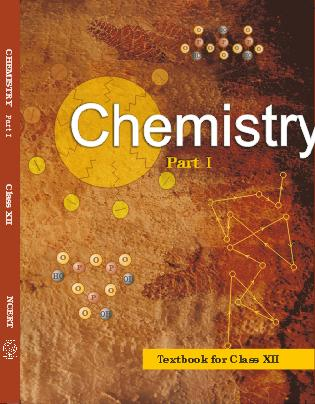## Class 12 Chemistry list of chapters

1. The Solid State
2. Solutions
3. Electrochemistry
4. Chemical Kinetics
5. Surface Chemistry
6. General Principles and Processes of Isolation of Elements
7. The p-Block Elements
8. The d and f Block Elements
9. Coordination Compounds
10. Haloalkanes and Haloarenes
11. Alcohols, Phenols and Ethers
12. Aldehydes, Ketones and Carboxylic Acids
13. Amines
14. Biomolecules
15. Polymers
16. Chemistry in Everyday life

## CBSE Question Paper 2009 class 12 Chemistry

General Instructions

1. All questions are compulsory.
2. Marks for each question are indicated against it.
3. Question numbers 1 to 8 are very short-answer questions, carrying 1 mark each. Answer these in one word or about one sentence each.
4. Question numbers 9 to 18 are short-answer questions, carrying 2 marks each. Answer these in about 30 words each.
5. Question numbers 19 to 27 are short-answer questions of 3 marks each. Answer these in about 40 words each.
6. Question numbers 28 to 30 are long-answer questions of 5 marks each. Answer these in about 70 words each.
7. Use Log Tables, if necessary Use of calculators is not permitted.

1. Which point defect in its crystal units alters the density of a solid? 

2. Why is the froth flotation method selected for the concentration of Sulphide ores? 

3. Define the term ‘Tyndall effect.’ 

4. Which is a stronger oxidizing agent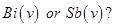5. Why is an alkylamine more basic than ammonia? 

6. Write the structure of 3 – oxopentanal. 

7. Give the IUPAC name of the following compound: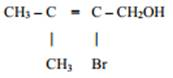8. Give an example of elastomers. 

9. Explain the role of 

(i)  Cryolite in the electrolytic reduction of alumina.

(ii)  Carbon monoxide in the purification of nickel.

10. A reaction is of second order with respect to a reactant. How will the rate of reaction 

(i)  doubled,

(ii)  Reduced to half?

11.  Differentiate between molality and molarity of solution.  What is the effect of change  in temperature of a solution on its molality and molarity? 

12. Draw the structures of the following molecules: 

(i)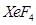(ii)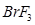13. Complete the following chemical reaction equations: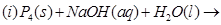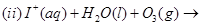14. Which ones in the following Pairs of substances undergoes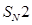substitution reaction faster and why?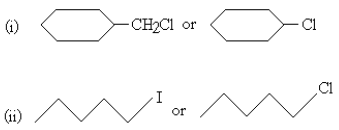15. Explain what is meant by 

16. Complete the following reaction equations: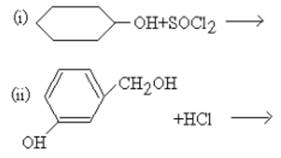17. Draw the structures of the monomers of the following polymers: 

(i)  Teflon

(ii)  Polythene

OR

What is the repeating unit in the condensation polymer obtained by combining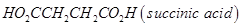and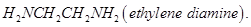18. Name two water soluble vitamins, their sources and the diseases caused due to their deficiency in diet. 

19.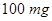of a protein is dissolved in just enough water to make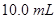of solution. If this solution has an osmotic pressure of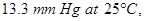what is the molar mass of the protein?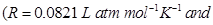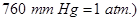20. Iron has a body centred cubic unit cell with a cell edge of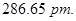The density of iron is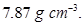Use this information to calculate Avogadro’s number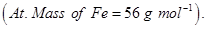21. What is the difference between multimolecular and macromolecular colloids? Give one example of each. How are associated colloids different from these two types of colloids? 

22. A first order reaction has a rate constant of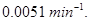If we begin with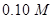concentration of the reactant, what concentration of reactant will remain in solution after 3 hours? 

23. For the complex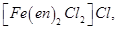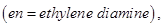identify 

(i) the oxidation number of iron,

(ii) the hybrid orbitals and the shape of the complex,

(iii) the magnetic behaviour of the complex,

(iv) the number of geometrical isomers,

(v) whether there is an optical isomer also, and

(vi) name of the complex, (At. no. of Fe = 26)

24. Explain the following observations: 

(i)  Fluorine does not exhibit any positive oxidation state.

(ii)  The majority of known noble gas compounds are those of Xenon.

(iii)  Phosphorus is much more reactive than nitrogen.

25. Giving an example for each describe the following reactions: 

(i)  Hofmann’s bromamide reaction

(ii)  Gatterman reaction

(iii)  A coupling reaction

26. Explain the mechanism of the following reactions: 

(i)  Addition of Grignard’s reagent to the carbonyl group of a compound forming an adduct followed by hydrolysis.

(ii)  Acid catalysed dehydration fan alcohol forming analkene.

(iii)  Acid catalysed hydration of an alkene forming an alcohol.

27. How do antiseptics differ from disinfectants? Give one example of each type. 

28. (a) Complete the following chemical reaction equations: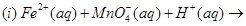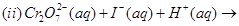(b) Explain the following observations:

(i) Transition elements are known to form many interstitial compounds.

(ii) With the same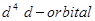configuration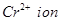is reducing while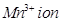is oxidising.

(iii) The enthalpies of atomization of the transition elements are quite high.

OR

(a) Complete the following chemical reaction equations: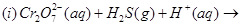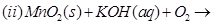(b) Explain the following observations:

(i) Transition metals form compounds which are usually coloured.

(ii) Transition metals exhibit variable oxidation states.

(iii) The actinoids exhibit a greater range of oxidation states than the lanthanoids.

29. (a) What type of a cell is the lead storage battery? Write the anode and the cathode reactions and the overall reaction occurring in a lead storage battery while operating. 

(b) A voltaic cell is set up at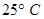with the half-cells,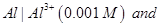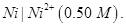Write the equation for the reaction that occurs when the cell generates an electric current and determine the cell potential.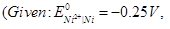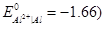OR

(a)  Express the relationship amongst cell constant, resistance of the solution in the cell and conductivity of the solution. How is molar conductivity of a solute related to conductivity of its solution?

(b)  Calculate the equilibrium constant for the reaction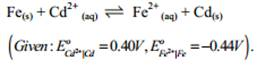30. (a) Illustrate the following name reactions by giving example: 

(i)  Cannizzaro’s reaction

(ii)  Clemmensen reduction

(b)  An  organic  compound  A  contains  69.77%  carbon,  11.63%  hydrogen  and  rest  oxygen.  The molecular  mass  of  the  compound  is  86.  It  does  not  reduce  Tollen’s  reagent  but  forms  and  addition compound with sodium hydrogen sulphite and gives positive iodoform test. On vigorous oxidation is ethanoic and propanoic acids. Derive the possible structure of compound A.

OR

(a) How are the following obtained?

(i) Benzoic acid from ethyl benzene.

(ii) Benzaldehyde from toluene.

(b) Complete each synthesis by giving the missing material, reagent or products: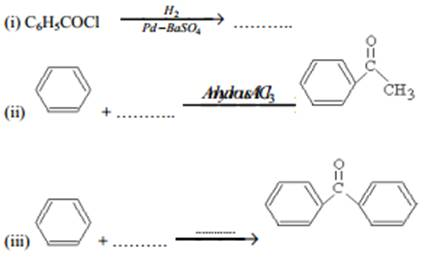These are questions only. To view and download complete question paper with solution install myCBSEguide App from google play store or login to our student dashboard.

## Chemistry Question Paper 2009

Download class 12 Chemistry question paper with solution from best CBSE App the myCBSEguide. CBSE class 12 Chemistry question paper 2009 in PDF format with solution will help you to understand the latest question paper pattern and marking scheme of the CBSE board examination. You will get to know the difficulty level of the question paper. CBSE question papers 2009 for class 12 Chemistry have 30 questions with solution.

## Previous Year Question Paper for class 12 in PDF

CBSE question papers 2018, 2017, 2016, 2015, 2014, 2013, 2012, 2011, 2010, 209, 2008, 2007, 2006, 2005 and so on for all the subjects are available under this download link. Practicing real question paper certainly helps students to get confidence and improve performance in weak areas.

To download CBSE Question Paper 2009 class 12 Accountancy, Chemistry, Physics, History, Political Science, Economics, Geography, Computer Science, Home Science, Accountancy, Business Studies and Home Science; do check myCBSEguide app or website. myCBSEguide provides sample papers with solution, test papers for chapter-wise practice, NCERT solutions, NCERT Exemplar solutions, quick revision notes for ready reference, CBSE guess papers and CBSE important question papers. Sample Paper all are made available through the best app for CBSE students and myCBSEguide website.